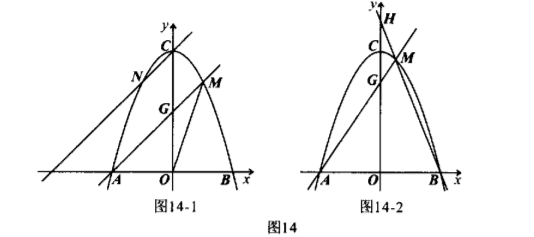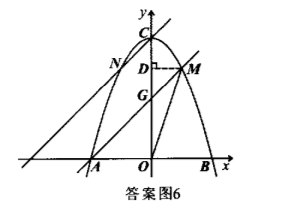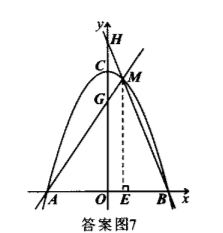（1）求该抛物线的解析式;
(2) 如图 14-1, $N$ 是抛物线上一点, 且位于第二象限, 连接 $O M$, 记 $\triangle A O G, \triangle M O G$ 的面积分别为 $S_1, S_2$. 当 $S_1=2 S_2$, 且直线 $C N / / A M$ 时, 求证: 点 $N$ 与点 $M$ 关于 $y$ 轴对称;
(3) 如图 14-2, 直线 $B M$ 与 $y$ 轴交于点 $H$, 是否存在点 $M$, 使得 $2 O H-O G=7$. 若 存在, 求出点 $M$ 的坐标; 若不存在, 请说明理由.【答案】 解: (1) $\because$ 抛物线 $y=a x^2+c$ 与 $x$ 轴交于点 $B(2,0)$, 顶点为 $C(0,4)$,
$\therefore\left\{\begin{array}{l}4 a+c=0, \\ c=4 .\end{array}\right.$ 解得 $\left\{\begin{array}{l}a=-1, \\ c=4 .\end{array}\right.$
$\therefore$ 该抛物线的解析式为 $y=-x^2+4$.
(2) 证明: 如答案图 6. 过点 $M$ 作 $M D \perp y$ 轴, 垂足为 $D$. 当 $\triangle A O G$ 与 $\triangle M O G$ 都以 $O G$ 为底时, $\because S_1=2 S_2, \therefore O A=2 M D$. 当 $y=0$ 时, 则 $-x^2+4=0$,

$\therefore O A=2, M D=1$. 设点 $M$ 的坐标为 $\left(m,-m^2+4\right)$,

$\because$ 点 $M$ 在第一象限, $\therefore m=1$,
$$\therefore-m^2+4=3, \therefore M(1,3) \text {. }$$

$$\therefore\left\{\begin{array} { l } { - 2 k _ { 1 } + b _ { 1 } = 0 , } \\ { k _ { 1 } + b _ { 1 } = 3 . } \end{array} \text { 解得 } \left\{\begin{array}{l} k_1=1, \\ b_1=2 . \end{array}\right.\right.$$
$\therefore$ 直线 $A M$ 的解析式为 $y=x+2$.

$\because$ 直线 $C N / / A M, \therefore k_2=k_1=1, \therefore y=x+b_2, \because C(0,4), \therefore b_2=4$.
$\therefore$ 直线 $C N$ 的解析式为 $y=x+4$, 将其代入 $y=-x^2+4$ 中,

$\because$ 点 $N$ 在第二象限, $\therefore$ 点 $N$ 的横坐标为 $-1, \therefore y=3, \therefore N(-1,3)$.
$\because M(1,3), \therefore$ 点 $N$ 与点 $M$ 关于 $y$ 轴对称（3）如答案图 7.

\begin{aligned} & \because M\left(m,-m^2+4\right), \therefore O E=m, M E=-m^2+4 \\ & \because B(2,0), \therefore O B=2, \quad \therefore B E=2-m . \end{aligned}

$$\because \tan \angle M B E=\tan \angle H B O, \quad \therefore \frac{E M}{B E}=\frac{O H}{B O},$$\begin{aligned} & \therefore O H=\frac{E M \cdot B O}{B E}=\frac{2\left(-m^2+4\right)}{2-m}=2(2+m)=2 m+4 . \\ & \because O A=2, \quad \therefore A E=m+2, \end{aligned}

\begin{aligned} & \therefore \frac{O G}{A O}=\frac{E M}{A E}, \quad \therefore O G=\frac{E M \cdot A O}{A E}=\frac{2\left(-m^2+4\right)}{m+2}=2(2-m)=4-2 m . \\ & \because 2 O H-O G=7, \quad \therefore 2(2 m+4)-(4-2 m)=7, \quad \therefore m=\frac{1}{2} . \end{aligned}

$\therefore$ 存在点 $M\left(\frac{1}{2}, \frac{15}{4}\right)$, 使得 $2 O H-O G=7 .$